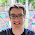## Friday, 15 April 2011

### NIALM as a combinatorial optimisation problem - continued

As previously discussed, appliance loads can be disaggregated from premise-level power readings by the minimisation of a function f at each time slice:

where:

• Pagg - premise-level aggregate power
• N - number of appliances
• I - vector of appliance binary indicators (1 if appliance is on, 0 otherwise)
• P - vector of appliance power when appliance is on
Two commonly cited disadvantages of this approach is how its accuracy would decrease with the number of appliances and level of noise. However, no research has been published investigating the situations in which this approach performs sufficiently accurately. I have tested this approach using simulated data for 24 time slices, relating to one reading per hour over the course of a day. Below are tests evaluating the performance of this approach as the number of appliances is increased and as the noise in the data is increased.

Increasing numbers of appliances

Below shows a graph of this approach's true positive rate (TPR) and false positive rate (FPR) for increasing numbers of appliances, n. No noise was introduced over the simulated data set.

We can see that this approach performs very accurately from n = 1 to roughly n = 10. However, for n > 10 the TPR rapidly decays towards 0.5, while the FPR increases towards 0.25. This approach is clearly only useful for small n (roughly n < 20). However, it might be interesting to evaluate the performance of this method given only a small subset of appliances and the premise-level aggregate power. The appliances should be chosen such that they are the most power hungry appliances.

Increasing noise

To investigate how the accuracy of this approach is affected by noise, Gaussian noise was added to the premise-level power readings of the form:
where:
• N - Gaussian distribution
• x - Noisy premise-level aggregate
• Pagg - actual premise-level aggregate
• c - noise level
Below shows a graph of this approach's true positive rate (TPR) and false positive rate (FPR) for increasing noise in the aggregate power reading, c.

We can see that instantly after introducing some noise, the TPR rate drops to roughly 0.7 for Gaussian noise of standard deviation just 0.1% of the aggregate power level. It is clear that this approach is not robust to even small levels of noise in the premise-level aggregate power readings, as would be likely in a realistic setting.

Extensions

Two extensions are discussed below which could increase the accuracy of this approach.

First, the assumption that an appliance has only one state for which it can draw power can be relaxed. Instead, we allow appliances to operate in one of a number of K states, each of which has a unique power value. The appliance state indicator variable would therefore be a binary vector taking the form of a 1-of-K coding scheme, such that:
and
The optimal appliance combination would therefore be given by minimising the function f at each time slice:

Second, the assumption that appliance states at each time slice are independent of each other can be relaxed. This allows us to take into account information of known appliance lengths in the form of constraints, e.g. 10 minutes < fridge_cycle_duration < 20 minutes. However, this would significantly increase the complexity of the optimisation problem by including the additional time dimension.

1.1.